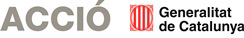# Fluid mechanics and aerodynamics

It is well known that approximate computational solutions are currently used to calculate the behavior of materials in any of their states, solid, liquid or gas, supporting such calculations with experimental tests and trails: In the case of fluids (i.e., calculations Aerodynamic) wind tunnel is used, and for solids (i.e., structural calculations), material tests. These calculation and testing operations make the resulting products much more expensive.

In this sense, the research effort we have made all these years has been rewarded with the discovery of a new aerodynamic lift theory, which we have included within the Geometric Theory of Fluids, about which we are writing a book. In these theories is explained precisely and mathematically demonstrates how aerodynamic lift and the resistance of an object submerged in a moving fluid happens, and how the phenomenon of turbulence develops.At the same time, several articles on fluid mechanics and, in particular, on the existence and regularity of the solutions of the Navier-Stokes equations are also being completed for publication in a mathematical journal, thus opting for a million dollars that grants the Clay Institute of Massachusetts.

Through these theories can be calculated much more accurately the behavior of fluids in any situation, without cost of computer hours or wind tunnel. As a research line, which we hope to develop during this year, we would like to mention the theory about deformable bodies, which among other things would allow to calculate both the resistance of materials and structural behavior, with a model that would also admit to incorporate other parameters. Finally, to point out that all these theories are intended to unify in a physical-mathematical theory of matter, which joins continuous media mechanics with thermo-dynamics, electromagnetism, gravitation and physics of matter, which would be the culmination of our work and would mean achieving one of the greatest challenges of physics.

PARTICIPATES